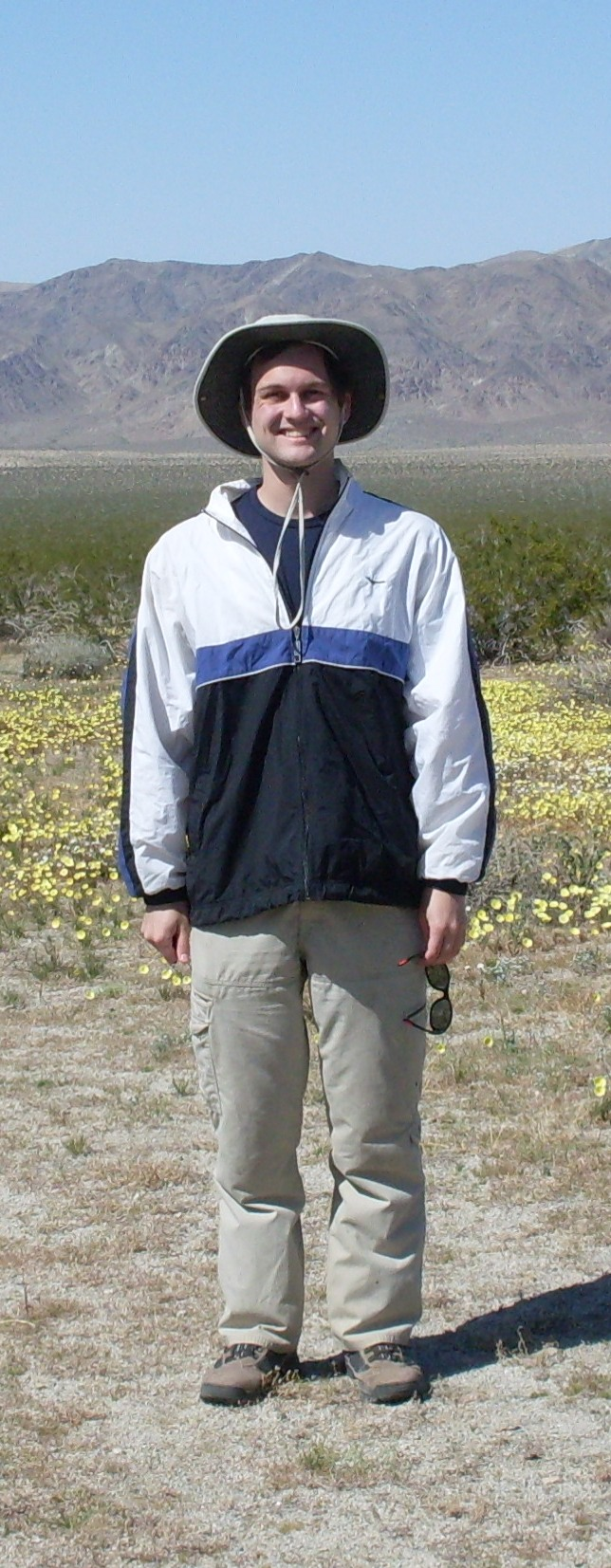My research is in the areas of geometric group theory and low-dimensional topology. Broadly speaking, I am interested in groups that arise naturally in geometry and topology and in ways that geometry and topology can be used to answer algebraic questions about groups. Most of my work has involved the notion of stable commutator length, a concept that has been the subject of a significant amount of recent interest. Stable commutator length can be studied from algebraic, topological, and functional analytic perspectives, and this contributes to its richness. My work on stable commutator length spans all of these perspectives.

My research is described in more detail in my research statement.

### Papers

Abstract: This paper has two parts, on Baumslag-Solitar groups and on general G-trees. In the first part we establish bounds for stable commutator length (scl) in Baumslag-Solitar groups. For a certain class of elements, we further show that scl is computable and takes rational values. We also determine exactly which of these elements admit extremal surfaces. In the second part we establish a universal lower bound of 1/12 for scl of suitable elements of any group acting on a tree. This is achieved by constructing efficient quasimorphisms. Calculations in the group BS(2,3) show that this is the best possible universal bound, thus answering a question of Calegari and Fujiwara. We also establish scl bounds for acylindrical tree actions. Returning to Baumslag-Solitar groups, we show that their scl spectra have a uniform gap: no element has scl in the interval (0, 1/12).

Immersed surfaces in the modular orbifold, with Danny Calegari, Proc. Amer. Math. Soc. 139 (2011), 2295-2308.

Abstract: A hyperbolic conjugacy class in the modular group PSL(2,Z) corresponds to a closed geodesic in the modular orbifold. Some of these geodesics virtually bound immersed surfaces, and some do not; the distinction is related to the polyhedral structure in the unit ball of the stable commutator length norm. We prove the following stability theorem: for every hyperbolic element of the modular group, the product of this element with a sufficiently large power of a parabolic element is represented by a geodesic that virtually bounds an immersed surface.

A new computation of the codimension sequence of the Grassmann algebra, with Adilson Eduardo Presoto and Alan Tarr, Rose-Hulman Undergraduate Mathematics Journal 6 (2005), no. 2

Abstract: Krakowski and Regev found a basis of polynomial identities satisfied by the Grassmann algebra over a field of characteristic 0 and described the exact structure of these relations in terms of the symmetric group. Using this, they found an upper bound for the the codimension sequence of the T-ideal of polynomial identities of the Grassmann algebra. Working with certain matrices, they found the same lower bound, thus determining the codimension sequence exactly. In this paper, we compute the codimension sequence of the Grassmann algebra directly from these matrices, thus obtaining a proof of the codimension result of Krakowski and Regev using only combinatorics and linear algebra. We also obtain a corollary from our proof.

Department of Mathematics
The University of Oklahoma
Norman, OK 73019-3103
(626) 200-9460
jlouwsma AT ou DOT edu
Physical Sciences Center 1007